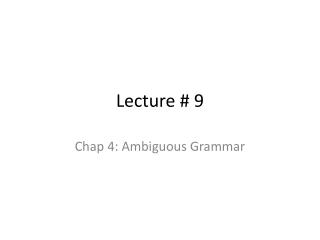DownloadDownload PresentationLecture # 9

# Lecture # 9

Télécharger la présentation## Lecture # 9

- - - - - - - - - - - - - - - - - - - - - - - - - - - E N D - - - - - - - - - - - - - - - - - - - - - - - - - - -
##### Presentation Transcript

1. Lecture # 9 Chap 4: Ambiguous Grammar

2. Chomsky Hierarchy: Language Classification • A grammar G is said to be • Regular if it is right linear where each production is of the formA  w B or A  wor left linear where each production is of the formA  B w or A  w • Context free if each production is of the formA  where A  N and   (NT)* • Context sensitive if each production is of the form  A     where A  N, ,,  (NT)*, || > 0 • Unrestricted

3. Chomsky Hierarchy L(regular)  L(context free)  L(context sensitive)  L(unrestricted) Where L(T) = { L(G) | G is of type T }That is: the set of all languagesgenerated by grammars G of type T Examples: Every finite language is regular! (construct a FSA for strings in L(G)) L1= { anbn | n 1 } is context free L2= { anbncn | n 1 } is context sensitive

4. Parse Trees • The root of the tree is labeled by the start symbol • Each leaf of the tree is labeled by a terminal (=token) or  • Each interior node is labeled by a nonterminal • If AX1 X2 … Xn is a production, then node A has immediate childrenX1, X2, …, Xn where Xi is a (non)terminal or  ( denotes the empty string)

5. Parse Tree for the Example Grammar Parse tree of the string 9-5+2 using grammar G list list digit list digit digit The sequence ofleafs is called theyield of the parse tree 9 - 5 + 2

6. Example of Parse Tree • Suppose we have the following grammar E → E + E E → E * E E → ( E ) E → - E E → id Perform Left most derivation, right most derivation and construct a parse tree for the string id+id*id

7. Two possible Parse Trees using Leftmost derivation

8. Parse Tree via Right most derivation

9. Ambiguity • Grammar is ambiguous if more than one parse tree is possible for some string as shown in the previous example. If there are more than one left most derivations or more than one right most derivations. • Ambiguity is not acceptable • Unfortunately, it’s undecidable to check whether a given CFG is ambiguous • Some CFLs are inherently ambiguous (do not have an unambiguous CFG)

10. Ambiguity (cont’d) Consider the following context-free grammar: G = <{string}, {+,-,0,1,2,3,4,5,6,7,8,9}, P, string> with production P = string string+string | string-string | 0 | 1 | … | 9 This grammar is ambiguous, because more than one parse treerepresents the string 9-5+2

11. Two Parse Trees for the same string string string string string string string string string string string 9 - 5 + 2 9 - 5 + 2

12. Practice • Show that the following grammar is ambiguous: (Find out strings and two parse trees) 1) S AB | aaB 2) S a | abSb |aAb A a | Aa A bS | aAAb Bb 3) S aSb | SS | ε

13. How to eliminate Ambiguity? • If ambiguity is of the form: S α S β S  | α1 |……| αn Rewrite: S α S β S’  | S’ S’ α1 |……| αn • Make the following grammar unambiguous: E E+E | E*E | (E) | id

14. Eliminate Left Recursion If S S α for some α then there exists left recursion If there are productions: S S α | β Rewrite: S β S’ S’ α S’ | 

15. Example • Remove Left Recursion: S A A Sd | b